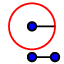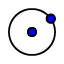# Circling The Drain

## Definition 15-18

A circle is a plane figure contained by one line such that all the straight lines falling upon it from one point among those lying within the figure equal one another. A circle is the figure contained by a curve such that every point on the curve is the same distance from a point in the figure. And the point is called the center of the circle. The boundary of a circle is a line, which is made of points (string of pearls), all the points on that line are the same distance from the center. This idea is what makes circles so important and useful in geometry because we can quickly mark lines and distances that are the same just using circles. A diameter of the circle is any straight line drawn through the center and terminated in both directions by the circumference of the circle, and such a straight line also bisects the circle. When we talk about diameters we really have two different definitions, one is a line that goes through the center of a circle and terminates on the circumference of the circle and the other is the length across the circle. Generally speaking in Euclidean Geometry, when we say diameter we mean the line definition since we are not usually allowed to measure anything, the length across the circle is not as important. With this comes the concept that circles have many diameters. If you draw a circle below then draw a diameter of that circle, is that the only one you can draw? No, you can draw many more in different orientations around the circle. We also have the concept of a radius to add here, which is any line with terminating points on the circumference of the circle and the center. All diameters in a give are congruent as are all radii in a circle with each other. A semicircle is the figure contained by the diameter and the circumference but off by it. And the center of the semicircle is the same as that of a circle. Fancy way of saying a semicircle is half a circle, one side is half the circumference of the circle and the other is a diameter of the same circle. Semi means half

## Tool Practice

We now have a new tool, the compassand the circle with a given center. If you start with thetool you can draw circles of various sizes, the first click marks the center of the circle and the second click puts a point on the edge of the circle where you click. Thetool cannot draw circles on its own but can be used as a way to cheat and measure objects in our figure by copying circles. Use thetool and click on the point you created on the edge of your circle, then click on the center of your circle, you now have a copy of that circle you can move around. This is useful when you want to draw the same circle in different places also. Play around with the tools and get used to using them!